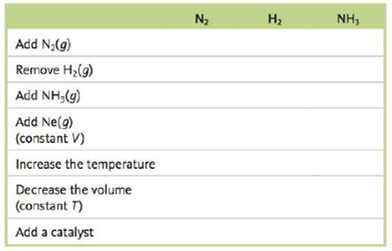Chapter 13, Problem 97CWP

Chapter
Section
Textbook Problem

# Consider the following exothermic reaction at equilibrium: N 2 ( g ) + 2 H 2 ( g ) ⇌ 2 NH 3 ( g ) Predict how the following changes affect the number of moles of each component of the system after equilibrium is reestablished by completing the table below. Complete the table with the terms increase, decrease, or no change.Interpretation Introduction

Interpretation: The given table is to be completed with the terms increase, decrease or no change in the number of moles.

Concept introduction: The equilibrium constant describes the ratio of the reactant to the product on the equilibrium conditions in terms of the concentrations.

Law of mass action is applicable on the equilibrium reactions.

Le Chatelier’s principle states that the change in any factor such as temperature, pressure, concentration and catalyst shifts the direction of equilibrium which nullifies the relative effect.

To determine: The changes which affect the number of moles of each component of the system after equilibrium reestablished by completing the given table.

Explanation

Explanation

Given

The reaction is given as,

N2(g)+3H2(g)2NH3(g)

This is an exothermic reaction at equilibrium.

As the Le Chatelier’s principle states that the equilibrium point shifts in the direction which relieves the stress. Therefore the following effects are observed in terms of the number of moles of reactants and product for the formation of Ammonia.

• Addition of reactant shifts the equilibrium reaction to the right and it increases the concentration of the product.
• Removal of reactant shifts the equilibrium reaction to the left and decreases the concentration of the product.
• Addition of product shifts the equilibrium reaction to the left and increases the concentration of the reactants.
• Addition of unreactive species like inert gases has no effect on the either of the reactants or products.
• In case of exothermic reaction an increase in temperature shifts the equilibrium to the left and hence it increases the concentration of the reactants.
• The decrease in the volume the increases the concentration of both reactants and products.
• Addition of catalyst does not show any effect on equilibrium concentrations as the work of catalyst is the increase the rate of reaction only without involving in the concentrations.

The completed table is given as follows.

### Still sussing out bartleby?

Check out a sample textbook solution.

See a sample solution

#### The Solution to Your Study Problems

Bartleby provides explanations to thousands of textbook problems written by our experts, many with advanced degrees!

Get Started